# String Hashing 如何工作？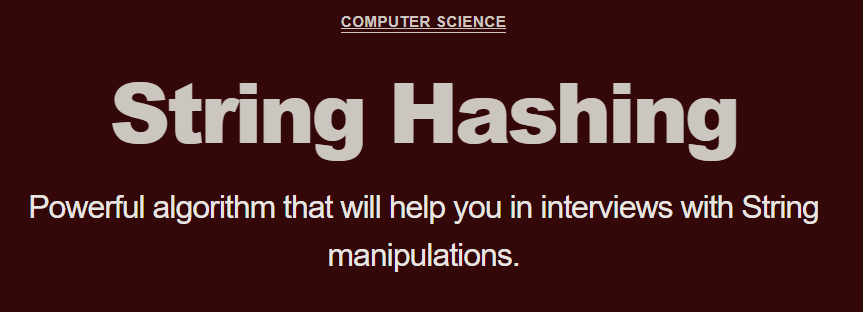``````const A = "imagine that this is a string of 200 characters"
const B = "imagine that this is a string of 200 characterz"
``````

``````const A = "imagine that this is a string of 200 characters"

const B = "imagine that this is a string of 200 characterz"

function equal(A, B) {
let i;
for(i = 0; i < A.length; i++){
if (A[i] !== B[i]) {
return false;
}
}
return true;
}

console.log(equal(A,B));
``````

``````function equal(A, B) {
if (A.lenght !== B.length) return false;
let i;
for(i = 0; i < A.length; i++){
if (A[i] !== B[i]) {
return false;
}
}
return true;
}
``````

# String Hash 公式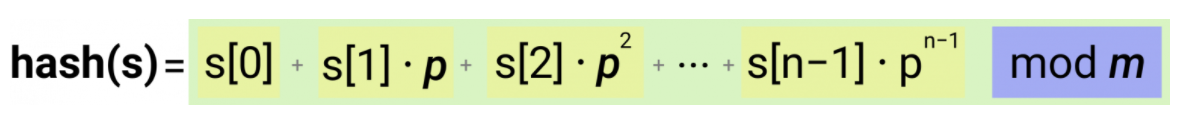p: 素数，大致等于所使用的不同字符的数量。例如，如果我们要计算一个只包括英文小写字母的单词的哈希值，31 会是个不错的数字。然而，如果我们还会使用大写字母的话，那么 53 是一个合适的选择。

m: 这个数字越大，两个随机字符串出现碰撞的概率就越小。这个变量也应该是素数。

10 ^ 9 + 9 是个常用的选择。

``````p = 31
m = 1e9 + 9
word = 'apple'
``````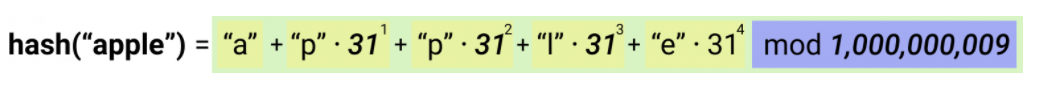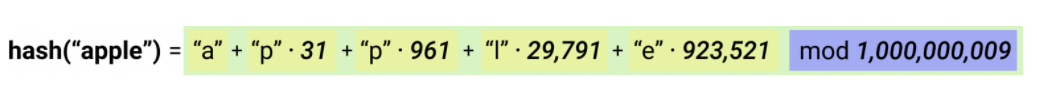``````"a" = 97
"p" = 112
"p" = 112
"l" = 108
"e" = 101
``````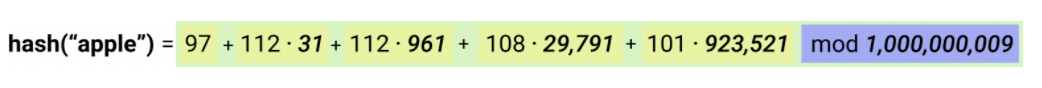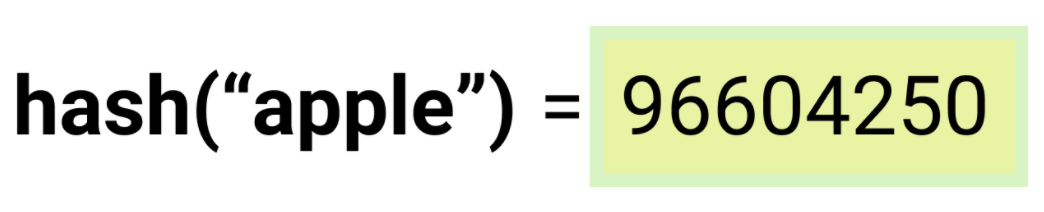``````function hash(word) {
var p = 31;
var m = 1e9 + 9;
var hash_value = 0;
for(var i = 0; i < word.length; i++) {
var letter = word[i];
var charCode = letter.charCodeAt();
hash_value = hash_value + (charCode * Math.pow(p, i))
}
return hash_value % m;
}

console.log(hash("apple"));
``````

This is a version from Jorge Chávez.